# DAV Class 6 Maths Chapter 8 Worksheet 1 Solutions

The DAV Class 6 Maths Solutions and DAV Class 6 Maths Chapter 8 Worksheet 1 Solutions of Basic Geometrical Concepts offer comprehensive answers to textbook questions.

## DAV Class 6 Maths Ch 8 WS 1 Solutions

Question 1.
Mark a point in your notebook and draw a line passing through it. How many lines can you draw passing through this point?
Steps:
(i) Take any point 0.
(ii) Draw a straight line through 0 making arrow on both ends.
(iii) Name the line $$\overrightarrow{\mathrm{PQ}}$$.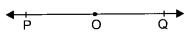Infinite number of lines can be drawn through this point.

Question 2.
Mark any two points in your notebook and draw a line passing through both the points. How many such lines can you draw passing through these two given points?
Steps:
(i) Take any two points A and B.
(ii) Put a scale at A and B and draw a line through A and B.
(iii) Extend it in both the directions making arrows on both ends.
(iv) AB is the required line.One and only one line can be drawn through two points.

Question 3.
Classify the following pairs of lines as parallel lines or intersecting lines.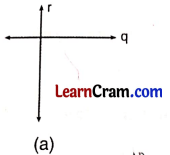IntersectingParallelParallel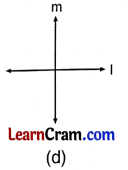Intersecting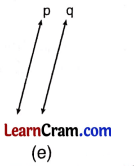Parallel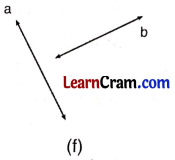IntersectingQuestion 4.
Fill in the blanks:
(a) An orange has a ________ surface.
Spherical

(b) A 100 rupee note has a ________ surface.
Plane

(c) ________ lines can pass through one given point in a plane.
Many

(d) Three or more points in a plane which lie on the same line are called ________.
Collinear

(e) Lines which do not meet anywhere in a plane are called ________ lines.
Parallel

(f) Two lines which cut each other at a point are called ________ lines.
Concurrent

Question 5.
In the given figure write:(a) all the pairs of parallel lines.
l ∥ m ∥ n

(b) the lines whose point of intersection is E.
n and r

(c) one set of 4 collinear points.
G, F, E, D and A, B, C, D

(d) any four pairs of intersecting lines
t and r, t and n, r and m, 1 and t

(e) the point of intersection of lines m and t.
B

(f) the point of intersection of lines r and t.
D

Question 6.
A, B, C are any three points in a plane. Join them in pairs. How many lines can you get if:
(a) A, B, C are collinear?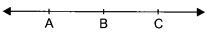Only one line is obtained when A, B, C are collinear.

(b) A, B, C are not collinear?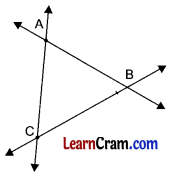Three fines $$\overrightarrow{\mathrm{AB}}, \overrightarrow{\mathrm{BC}}$$ and $$\overleftrightarrow{\mathrm{AC}}$$ can be obtained if A, B, C are not collinear.Question 7.
Lines l, m and n are concurrent. Also lines r, l and m are concurrent. Draw a figure and state whether lines r, l, m and n are concurrent or not.
Yes, the lines r, l, m and n are concurrent.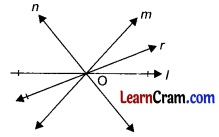Question 8.
In the given figure, name:(a) the lines concurrent at point A.
r, n and m

(b) all the sets of collinear points.
(A, F, B), (B, D, C), (A, E, C)

(c) the lines concurrent at point G.
r, q and p

(d) the point of intersection of three fines l, p and m.
C

(e) all the points that lie in this plane.
A, F, B, D, C, E and G

(f) two pairs of intersecting lines.
q and n, r and p

Question 9.
In the given figure name the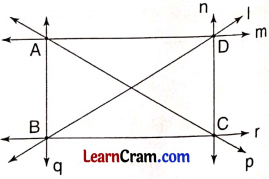(a) lines concurrent at D and B
(b) point of concurrence of fines AD, AB and AC.
(a) Lines concurrent at D are n, l and m.
(b) Point of concurrence of fines AD, AB and AC is A.

### DAV Class 6 Maths Chapter 8 Worksheet 1 Notes

Line: A straight path extendable endlessly in both the directions is called line.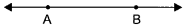$$\overline{\mathrm{AB}}$$ is the representation of line AB

Ray: Ray has one end point and extendable is one direction.$$\overline{\mathrm{AB}}$$ represents a ray

Line segment: Line segment is a part of a line. It has two end points and definite length.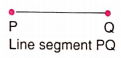Plane: Plane is a flat surface which can be extended infinitely in all directions.

Collinear points: The points which lie on the same line are called collinear points.Here P, Q, R are collinear points.

Non-collinear points:
The points which do not he on the same straight line are called non-collinear points.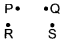Here P, Q, R, S are non collinear points.

Intersecting lines: The lines which cut. each other at. a point are called intersecting lines.Here lines $$\overrightarrow{\mathrm{AB}}$$ and $$\overleftrightarrow{\mathrm{CD}}$$ intersect at O.
Infinite number of lines can pass through a point.

Parallel lines:
The lines which never intersect each other even on extending infinitely in both directions are calLed parallel lines.
Here $$\overleftrightarrow{\mathrm{AB}} \| \overleftrightarrow{\mathrm{CD}}$$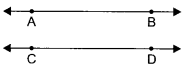Concurrent lines:
The lines which pass through the same point are called concurrent lines.
Here all the lines pass through the same point O.